# Edexcel A Level Chemistry:复习笔记2.1.4 Ionic Equations

### Writing Chemical Formulae

• Oxidation numbers are a useful tool for naming compounds as some elements can exist with more than one oxidation number
• For compound with two elements it is straight forward to name the compound
• For example
• PCl3 is phosphorus(III) chloride or phosphorus trichloride
• PCl5 is phosphorus(V) chloride or phosphorus pentachloride
• OF2 is oxygen difluoride
• O2F2 is dioxygen difluoride
• In order to name a more complete compound we use Roman numerals for the element that has a variable oxidation number
• K2CrO4 potassium chromate(VI)

#### Worked Example

Can you name these metal compounds?

1. Cu2O
2. MnSO4
3. Na2CrO4
4. KMnO4
5. Na2Cr2O7

Answer 1: copper(I) oxide:

The ox. no. of 1 O atom is -2 and Cu2O has overall no charge so the ox. no. of Cu is +1

Answer 2: manganese(II) sulfate:

The charge on the sulfate ion is -2, so the charge on Mn and ox. no. is +2

Answer 3: sodium chromate(VI):

The ox. no. of 2 Na atoms is +2 so CrO4 has an overall -2 charge, so the ox. no. of Cr is +6

Answer 4: potassium manganate(VII):

The ox. no. of a K atom is +1 so MnO4 has overall -1 charge, so the ox. no. of Mn is +7

Answer 5: sodium dichromate(VI):

The ox. no. of 2 Na atoms is +2 so Cr2O7 has an overall -2 charge, so the ox. no. of Cr is +6. To distinguish it from CrO4 we use the prefix di in front of the anion

### Ionic Half-Equations

#### Balancing full ionic equations

• Balancing equations using redox principles is a useful skill and is best illustrated by following an example
• It is important to follow a methodical step-by-step approach so that you don't get lost:

#### Worked Example

Writing overall redox reactions

Manganate(VII) ions (MnO4- ) react with Fe2+ ions in the presence of acid (H+) to form Mn2+ ions, Fe3+ ions and water

Write the overall redox equation for this reaction

Step 1: Write the unbalanced equation and identify the atoms which change in oxidation number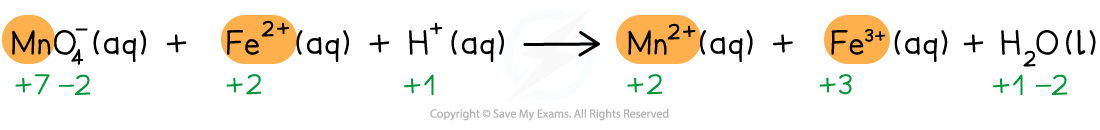Step 2: Deduce the oxidation number changes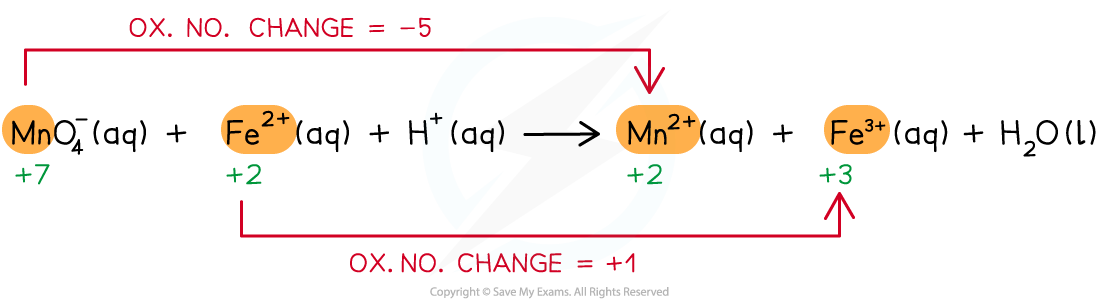Step 3: Balance the oxidation number changes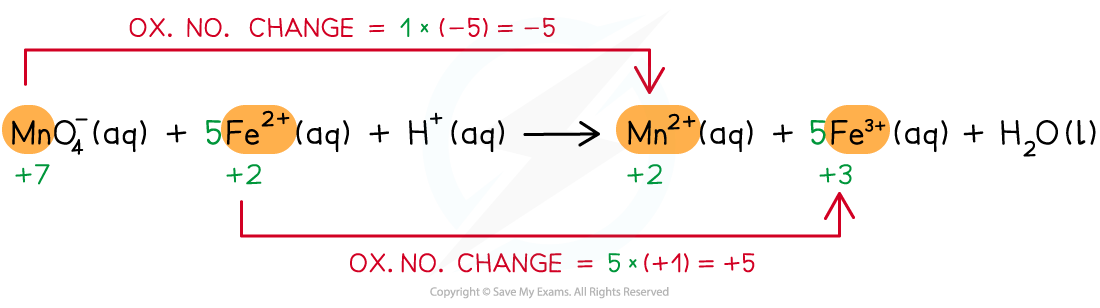Step 4: Balance the charges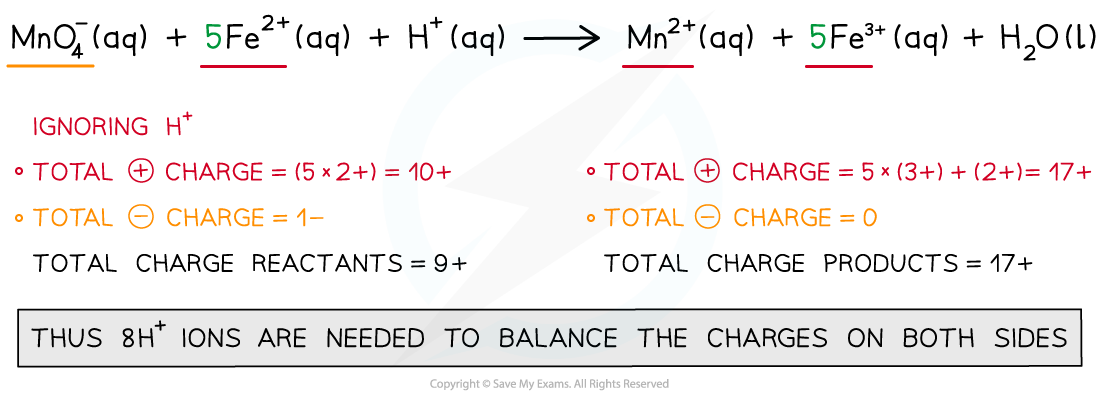Step 5: Finally, balance the atoms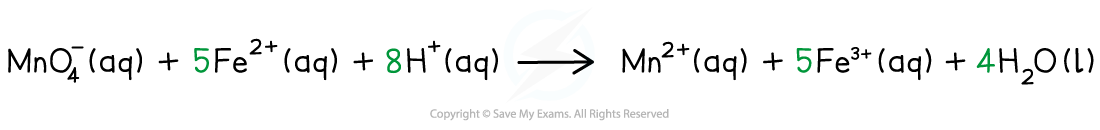### Metals & Non-metals

#### Metals

• Metals, in general, will form positive ions by losing electrons
• Therefore, they are oxidised and the oxidation number increases
• Example 1:
• When sodium reacts with water, sodium hydroxide and hydrogen gas is formed

2Na (s) + H2O (l) → 2NaOH (aq) + H2 (g)

• The oxidation number of sodium changes from 0 to +1
• Example 2:
• When magnesium reacts with hydrochloric acid, magnesium chloride and hydrogen gas is formed

Mg (s) + 2HCl (l) → MgCl2 (aq) + H2 (g)

• The oxidation number of magnesium changed from 0 to +2

#### Non-metals

• Non-metals, in general, will form negative ions by gaining electrons
• Therefore, they are reduced and the oxidation number decreases
• Example:
• When sodium reacts with oxygen, sodium oxide is formed

4Na (s) + O2 (g) → Na2O (s)

• The oxidation number of oxygen changes from 0 to -2